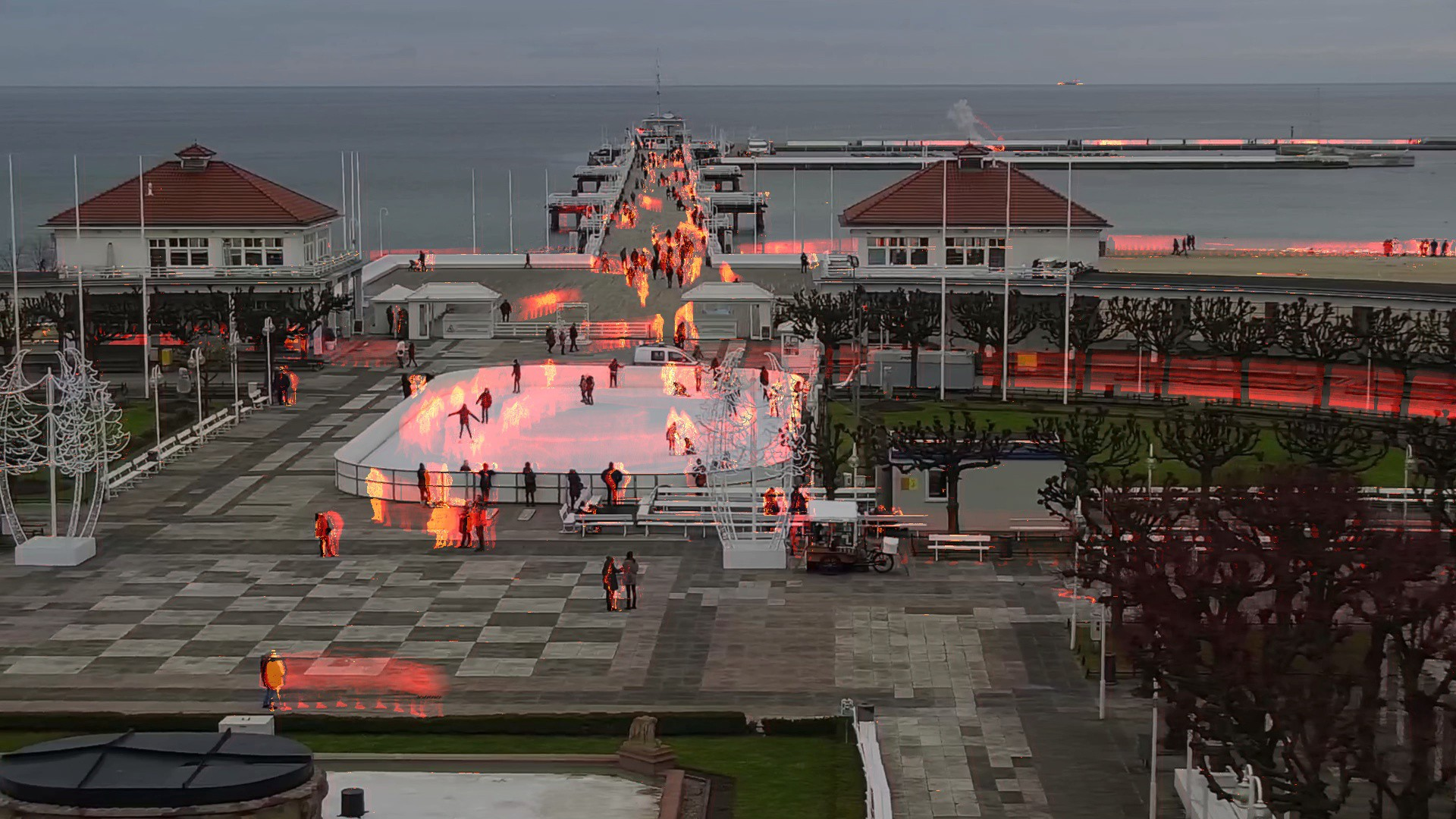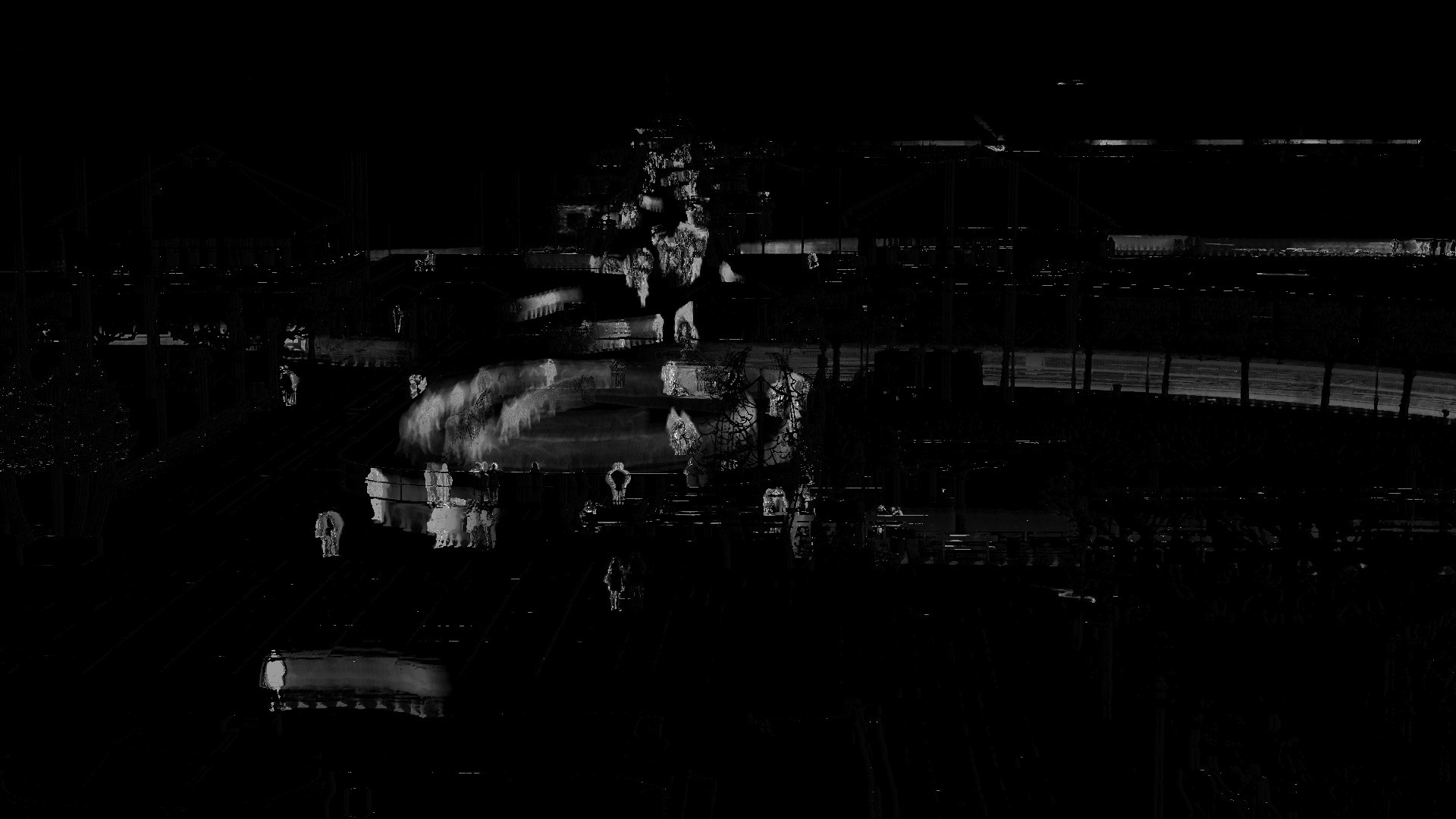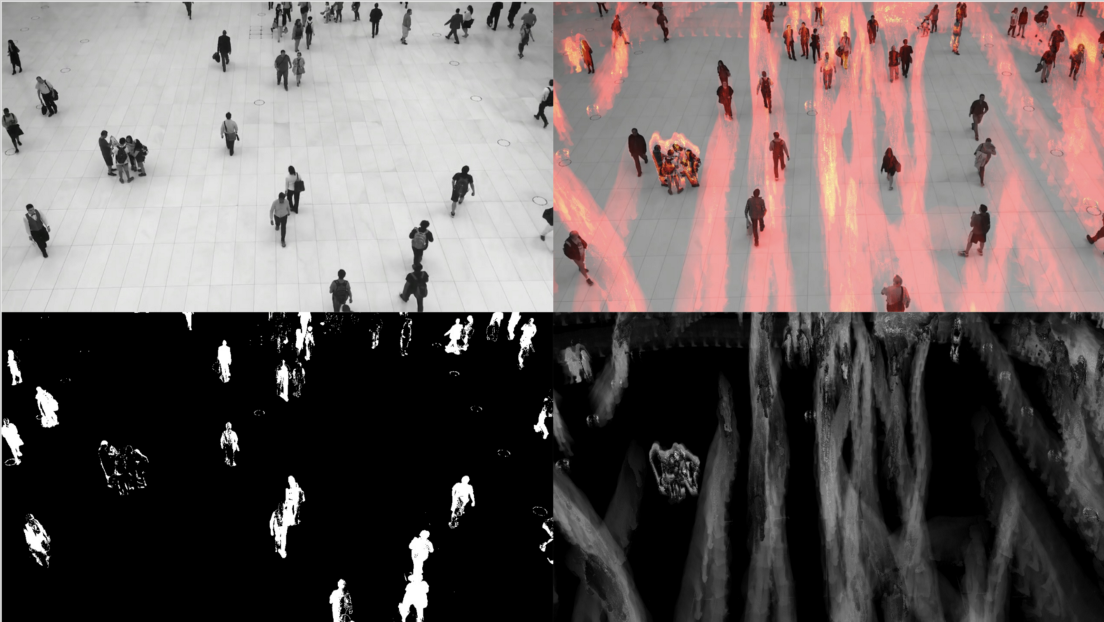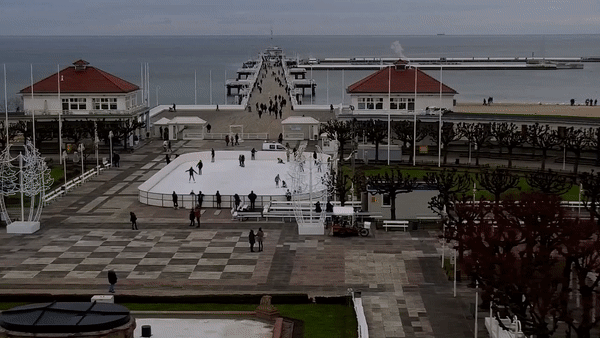# 使用OpenCV和Python构建运动热图视频

• 时间:

OpenCV是一个强大的图像和视频处理库，在这篇文章中，我将创建一个运动热图，用于检测运动、一些物体或人的流动方向，以及在投影公共区域时对建筑师的帮助。# 简介：

OpenCV，或（开源计算机视觉）是英特尔于1999年开发的一个库，主要是计算机视觉和实时视频操作，它是用C++编写的，但受不同的语言（包括Python）支持。

# 工作流程：

• 背景初始化：在第一步中，通过冻结第一帧来计算背景的模型。
• 更新：在第二步中，下一帧将从上一帧中减去，因此，如果两帧之间发生变化（移动），则这些帧的差异将反映出该变化，可以通过应用过滤器来进行市场销售。# 代码：

• https://github.com/robertosannazzaro/motion-heatmap-opencv/blob/master/README.md

``````capture = cv2.VideoCapture('input.mp4')
background_subtractor = cv2.bgsegm.createBackgroundSubtractorMOG()
length = int(capture.get(cv2.CAP_PROP_FRAME_COUNT))
``````

``````for i in range(0, length):

ret, frame = capture.read()

# If first frame
if first_iteration_indicator == 1:

first_frame = copy.deepcopy(frame)
height, width = frame.shape[:2]
accum_image = np.zeros((height, width), np.uint8)
``````

``````filter = background_subtractor.apply(frame)  # remove the background

threshold = 2
maxValue = 2
ret, th1 = cv2.threshold(filter, threshold, maxValue, cv2.THRESH_BINARY)

accum_image = cv2.add(accum_image, th1)

color_image_video = cv2.applyColorMap(accum_image, cv2.COLORMAP_HOT)
````````````video = cv2.VideoWriter('output.avi', fourcc, 30.0, (width, height))
for image in images:
video.write(cv2.imread(os.path.join(image_folder, image)))

cv2.destroyAllWindows()
``````